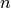# scipy.spatial.distance.seuclidean¶

scipy.spatial.distance.seuclidean(u, v, V)

Returns the standardized Euclidean distance between two n-vectors u and v. V is an n-dimensional vector of component variances. It is usually computed among a larger collection vectors.

Parameters : u : ndarray An-dimensional vector. v : ndarray An-dimensional vector. V : ndarray An-dimensional vector. d : double The standardized Euclidean distance between vectors u and v.

#### Previous topic

scipy.spatial.distance.russellrao

#### Next topic

scipy.spatial.distance.sokalmichener